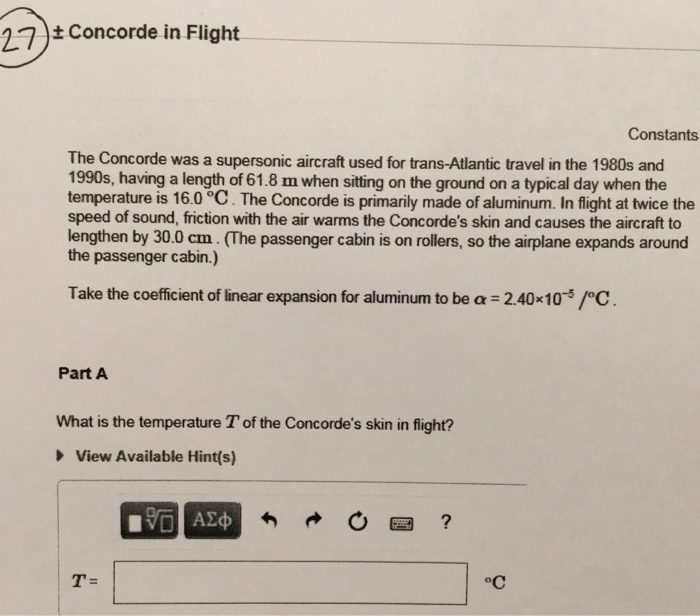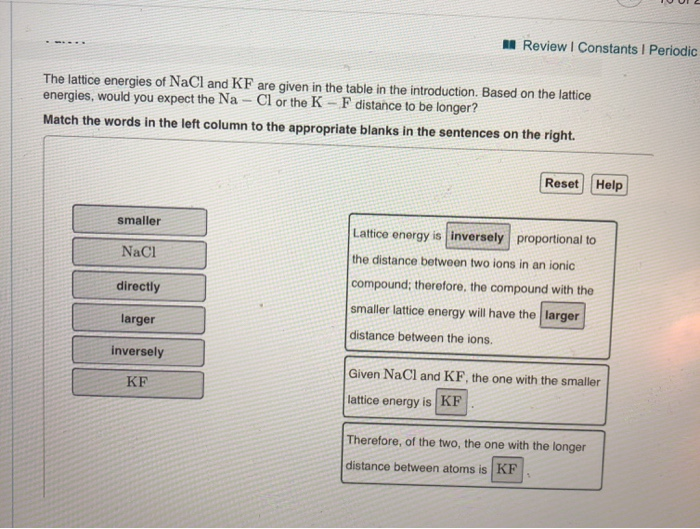Question

explain how time of flight is used to measure lattice constants

Explain how time of flight is used to measure lattice constants

The lattice constant is actually dimension of unit cells in a crystal lattice. Three dimenions are a, b, and c. in case of cubic constants are equal to a only

generally, ToF is a method used to measure the distance between a sensor and an object (or lattice plane), when signal emmited from sensor reflected by an object based on the time taken by signal. The total time taken from senor to target and target to sensor is time of flight.

so distance is=time of flight/2 * velocity of signal

generally x ray are used for lattice dimension determination with and robust algorithm for higher accuracy.

#### Earn Coins

Coins can be redeemed for fabulous gifts.

Similar Homework Help Questions
• ### 27 Concorde in Flight Constants The Concorde was a supersonic aircraft used for trans-Atlantic travel in...27 Concorde in Flight Constants The Concorde was a supersonic aircraft used for trans-Atlantic travel in the 1980s and 1990s, having a length of 61.8 m when sitting on the ground on a typical day when the temperature is 16.0 °C. The Concorde is primarily made of aluminum. In flight at twice the speed of sound, friction with the air warms the Concorde's skin and causes the aircraft to lengthen by 30.0 cm. (The passenger cabin is on rollers, so...

• ### 6. Explain how the Doppler effect is used by the police to measure the speed of...

6. Explain how the Doppler effect is used by the police to measure the speed of a car.

• ### An acceleration voltage of 30.0 kV is used to accelerate ions into the 1.54 m flight tube of a time‑of‑flight (TOF) mass...

An acceleration voltage of 30.0 kV is used to accelerate ions into the 1.54 m flight tube of a time‑of‑flight (TOF) mass spectrometer. The frequency at which spectra can be recorded is dependent on how long it takes the slowest ion to travel from the source to the detector. If you wish to scan up to ?/?m/z 6801 at what frequency (spectra/s) could spectra be recorded if a new spectrum begins each time the slowest ion reaches the detector? frequency:................spectra/s

• ### Describe how variance and standard deviation are used to measure the variability of individual stocks. Explain...

Describe how variance and standard deviation are used to measure the variability of individual stocks. Explain how an investor chooses the best portfolio of stock to hold.

• ### What is the Born-Haber cycle? How is it used to determine lattice energy and how is...

What is the Born-Haber cycle? How is it used to determine lattice energy and how is Hess’s law used? Please write it all out, illustrate if necessary.

• ### Evaluate number o independent elastic constants for orthorhombic and tetragoal lattice systems.

Evaluate number o independent elastic constants for orthorhombic and tetragoal lattice systems.

• ### Explain briefly how gas chromatography and mass spectrometry can be used to identify and quantitavely measure...

Explain briefly how gas chromatography and mass spectrometry can be used to identify and quantitavely measure the components of an unk sample.

• ### flight time

Delta Airlines quotes a flight time of 2 hours, 5 minutes for its flights from Cincinnati to Tampa. Suppose we believe that actual flight timesare uniformly distributed between 2 hours and 2 hours, 20 minutes.1.a. What is the probability that the flight will be no more than 5 minuteslate?b. What is the probability that the flight will be more than 10 minutes late?c. What is the expected flight time, in minutes?1 is2.

• ### Calculate the probability that a flight will depart early or on-time. P(flight early/on time DEPP)=(#flights early/on...

Calculate the probability that a flight will depart early or on-time. P(flight early/on time DEPP)=(#flights early/on time)/(total # flights)=363/500= 0.726 Calculate the probability that a flight will arrive late. P(Late ARR)=(#flights late)/(total # flights)=170/500= 0.34 Calculate the probability that a flight departs late or arrives early (does not say on time). P(Late/early DEPP)=(#flights Late+ early)/(total # flights)=428/500= 0.856 If the above is correct, Assume now that the random variable X=Arrival Time is exactly normally distributed with mean m= -2.5 and...

• ### M Review | Constants 1 Periodic The lattice energies of NaCl and KF are given in...M Review | Constants 1 Periodic The lattice energies of NaCl and KF are given in the table in the introduction. Based on the lattice energies, would you expect the Na - Cl or the K - F distance to be longer? Match the words in the left column to the appropriate blanks in the sentences on the right. Reset Help smaller NaCl Lattice energy is inversely proportional to the distance between two ions in an ionic compound; therefore, the...